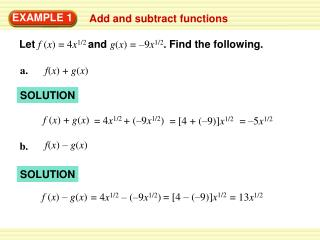Download PresentationSOLUTION

# SOLUTION - PowerPoint PPT PresentationDownload Presentation## SOLUTION

- - - - - - - - - - - - - - - - - - - - - - - - - - - E N D - - - - - - - - - - - - - - - - - - - - - - - - - - -
##### Presentation Transcript

1. a. f(x) + g(x) f(x) – g(x) b. EXAMPLE 1 Add and subtract functions Letf (x)= 4x1/2andg(x)=–9x1/2. Find the following. SOLUTION f (x) + g(x) = 4x1/2 + (–9x1/2) = [4 + (–9)]x1/2 = –5x1/2 SOLUTION f (x) – g(x) = [4 – (–9)]x1/2 = 13x1/2 = 4x1/2 – (–9x1/2)

2. The functions fand geach have the same domain: all nonnegative real numbers. So, the domains of f + gand f – galso consist of all nonnegative real numbers. c. the domains of f + gand f – g EXAMPLE 1 Add and subtract functions SOLUTION

3. a. f (x) g(x) b. 6x f (x) f (x) g(x) g(x) x3/4 f (x) g(x) = = 6x1/4 = 6x(1 – 3/4) EXAMPLE 2 Multiply and divide functions Let f (x)= 6xand g(x) = x3/4. Find the following. SOLUTION = (6x)(x3/4) = 6x(1 + 3/4) = 6x7/4 SOLUTION

4. The domain of f consists of all real numbers, and the domain of gconsists of all nonnegative real numbers. So, the domain of f gconsists of all nonnegative real numbers. Because g(0) = 0, the domain of is restricted to all positive real numbers. f g EXAMPLE 2 Multiply and divide functions f g and the domains of f c. g SOLUTION

5. r(m) s(m) (6 106)m0.2 = 241m–0.25 = • Findr(m) s(m). EXAMPLE 3 Solve a multi-step problem Rhinos For a white rhino, heart rate r(in beats per minute) and life span s(in minutes) are related to body mass m(in kilograms) by these functions: • Explain what this product represents.

6. Find and simplify r(m) s(m). r(m) s(m) = 241m –0.25 [ (6 106)m0.2 ] 241(6 106)m(–0.25 + 0.2) = (1446 106)m –0.05 = (1.446 109)m –0.05 = EXAMPLE 3 Solve a multi-step problem SOLUTION STEP 1 Write product of r(m) and s(m). Product of powers property Simplify. Use scientific notation.

7. Interpret r(m) s(m). EXAMPLE 3 Solve a multi-step problem STEP 2 Multiplying heart rate by life span gives the total number of heartbeats for a white rhino over its entire lifetime.

8. f (x) + g(x) f (x) – g(x) for Examples 1, 2, and 3 GUIDED PRACTICE Let f (x) = –2x2/3andg(x) = 7x2/3. Find the following. SOLUTION f (x) + g(x) = –2x2/3 + 7x2/3 = 5x2/3 = (–2 + 7)x2/3 SOLUTION f (x) – g(x) = –2x2/3 – 7x2/3 = [–2 + ( –7)]x2/3 = –9x2/3

9. the domains of f + gand f – g for Examples 1, 2, and 3 GUIDED PRACTICE SOLUTION The domains of f and g have the same domain: all non-negative real numbers. So , the domain of f + g and f – g also consist of all non-negative real numbers.

10. f (x) g(x) f (x) g(x) = 3x x1/5 f (x) g(x) f (x) 3x = g(x) x1/5 for Examples 1, 2, and 3 GUIDED PRACTICE Let f (x) = 3xandg(x) = x1/5. Find the following. SOLUTION = 3(x ) 1 + 1/5 =3x6/5 SOLUTION = 3(x ) 1 – 1/5 =3x4/5

11. the domains off g and SOLUTION The domain of f • g consists of all real numbers. The domain of consists of all real numbers except x = 0. f f g g for Examples 1, 2, and 3 GUIDED PRACTICE

12. Use the result of Example 3 to find a white rhino’s number of heartbeats over its lifetime if its body mass is 1.7 105kilograms. ANSWER about 7.92 108 heartbeats for Examples 1, 2, and 3 GUIDED PRACTICE Rhinos